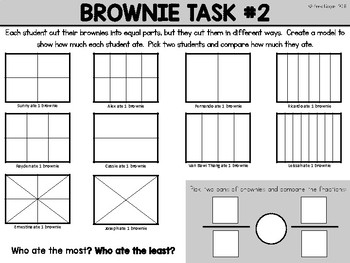# Math Center: Compare FractionsSubject
Resource Type
File Type

PDF

(7 MB|4 pages)
Product Rating
4.0
(1 Rating)
Standards
• Product Description
• StandardsNEW
Use this math task to to help students visualize equal parts of a whole. Students will shade the models to show how many brownies each student ate. Students will pick two brownie pans and compare the amount that each student ate. Task can be done multiple times in order to help students gain mastery.
Partition circles and rectangles into two, three, or four equal shares, describe the shares using the words halves, thirds, half of, a third of, etc., and describe the whole as two halves, three thirds, four fourths. Recognize that equal shares of identical wholes need not have the same shape.
Partition a rectangle into rows and columns of same-size squares and count to find the total number of them.
Compare two fractions with different numerators and different denominators, e.g., by creating common denominators or numerators, or by comparing to a benchmark fraction such as 1/2. Recognize that comparisons are valid only when the two fractions refer to the same whole. Record the results of comparisons with symbols >, =, or <, and justify the conclusions, e.g., by using a visual fraction model.
Explain why a fraction 𝘢/𝘣 is equivalent to a fraction (𝘯 × 𝘢)/(𝘯 × 𝘣) by using visual fraction models, with attention to how the number and size of the parts differ even though the two fractions themselves are the same size. Use this principle to recognize and generate equivalent fractions.
Explain equivalence of fractions in special cases, and compare fractions by reasoning about their size.
Total Pages
4 pages
N/A
Teaching Duration
N/A
Report this Resource to TpT
Reported resources will be reviewed by our team. Report this resource to let us know if this resource violates TpT’s content guidelines.